JEE  >  Theory & Procedure, Resonance Column

# Theory & Procedure, Resonance Column Notes - JEE

## Document Description: Theory & Procedure, Resonance Column for JEE 2022 is part of JEE preparation. The notes and questions for Theory & Procedure, Resonance Column have been prepared according to the JEE exam syllabus. Information about Theory & Procedure, Resonance Column covers topics like and Theory & Procedure, Resonance Column Example, for JEE 2022 Exam. Find important definitions, questions, notes, meanings, examples, exercises and tests below for Theory & Procedure, Resonance Column.

Introduction of Theory & Procedure, Resonance Column in English is available as part of our JEE preparation & Theory & Procedure, Resonance Column in Hindi for JEE courses. Download more important topics, notes, lectures and mock test series for JEE Exam by signing up for free. JEE: Theory & Procedure, Resonance Column Notes - JEE
 1 Crore+ students have signed up on EduRev. Have you?

Objective

To find the velocity of sound in air at room temperature using the resonance column by determining two resonance positions. Also find the velocity of sound in air at 00C.

Theory

Stationary waves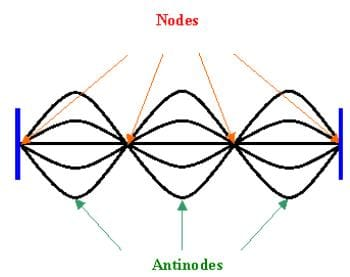Stationary waves are produced by the superposition of two waves of same frequency and amplitude travelling with same velocity in opposite directions. Due to the constructive interference, these waves produce certain fixed points along the medium which undergo zero displacement. These points of no displacement are known as nodes. Midway between every nodes are regions of maximum displacement. These points are called antinodes.

Production of longitudinal stationary waves in air column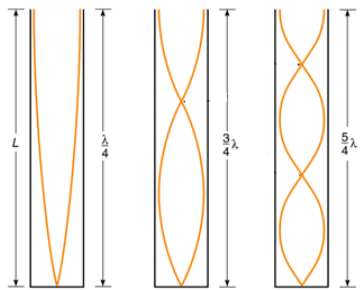Standing waves can be formed in a tube of air due to the interference of longitudinal sound waves travelling in opposite directions. In a pipe closed at one end, the closed end is a displacement node and the open end is a displacement antinode.

About Resonance column apparatus

Vibration of air column can be set up in a resonance column apparatus. It consists of a long metal tube held vertically in a tall jar containing the water. The tube can be fixed in vertical position. The length of the air column can be varied by raising or lowering the tube.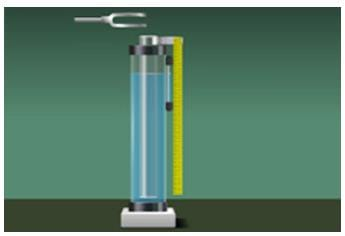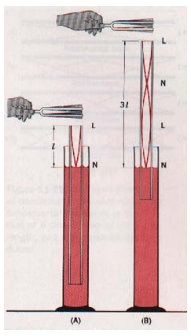Here, the surface of water will act as the closed end. When a vibrated tuning fork is held above the open end, longitudinal waves are sent down the air column. These waves are reflected at the water surface and thus produce standing waves. Nodes are produced at the water surface and antinodes are produced at the open end.

When the frequency of waves in the air column becomes equal to the natural frequency of tuning fork, a loud sound is produced in the air column. It is the condition for resonance. It occurs only when the length of air column is proportional to one-fourth of the wavelength of sound waves having frequency equal to frequency of tuning fork.

ie;   For first resonance,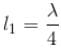For second resonance,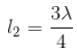From the above equations we get the relation,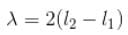Thus the velocity of sound waves on air at room temperature can be found as,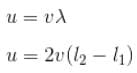ie;    which can be calculated.

If v be the velocity of sound in air at temperature t°C, then the velocity of sound at 0°C can be calculated as,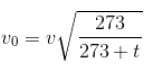Learning Outcomes

• Students understand the concept of standing waves and the various terms related to them.
• Students learn the relation between frequency, wavelength and velocity of a wave.
• Students understand transverse and longitudinal waves.

Materials Required

• Resonance column apparatus
• Tuning forks of known frequency
• Rubber hammer

Real Lab Procedure

• The inner tube of the resonance column is lowered to minimum so that the length of air column inside the tube is very small.
• Take a tuning fork of known frequency and striking it with a rubber hammer. The tuning fork now produces longitudinal waves with a frequency equal to the natural frequency of the tuning fork.
• Place the vibrating tuning fork horizontally above the tube. Sound waves are passes down to the tube and reflect back at the water surface.
• Now, raise the tube and the tuning fork until a maximum sound is heard.
• Measure the length of air column at that position. This is taken as the first resonant length, l1.
• Then raise the tube approximately about three times the first resonant length.
• Excite the tuning fork again and place it on the mouth of the tube.
• Change the height of the tube until the maximum sound is heard.
• Measure the length of air column at that position. This is taken as the second resonant length, l2.
• We can now calculate the velocity of sound in air at room temperature by using the relation,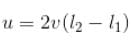• Repeat the experiment with tuning forks of different frequencies and in each time, calculate the value of v.
• The mean of the calculated values will give the velocity of sound in air at room temperature.
• By using the value of v, we can calculate the velocity of sound in air at 00C as,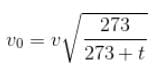Where,  v0 - velocity of sound in air at 00C.
v  - velocity of sound in air at temperature t.
t  - Room temperature.

Simulator Procedure (as performed through the Online Labs)

• Select the surrounding from the drop down list.
• Select the surrounding temperature using the slider.
• Select the diameter of the tube using the slider.
• Select the frequency of the tuning fork using the slider.
• Click on the ‘Hit tuning fork’ button to start/stop vibration.
• Click on the ‘Sound On’ button to hear the sound.
• Drag the inner tube/Change the slider to adjust the height of the air column
• Drag the apparatus to move it down.
• Click on the zoom in icon to view the zoomed area.
• Click on the ‘Show result’ button to see the result.
• To redo the experiment, click on the ‘Reset’ button.

Observations

 No. Frequency of tuning fork, ν (Hz) First resonating length, l1 (cm) Second resonating length, l2 (cm) l2-l1(×10-2cm) Velocity,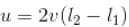(m/s) 1 2 Mean 1 2 Mean 1 2 3 4 5

Calculations

Room temperature, t  = ............. 0C

Velocity of sound in air at room temperature, v = ………... m/s

Velocity of sound in air at 00C,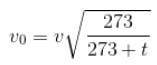= ............. m/s

Result

Velocity of sound in air at room temperature, v =……....... m/s

The document Theory & Procedure, Resonance Column Notes - JEE is a part of JEE category.
All you need of JEE at this link: JEE
 Use Code STAYHOME200 and get INR 200 additional OFF

Track your progress, build streaks, highlight & save important lessons and more!

,

,

,

,

,

,

,

,

,

,

,

,

,

,

,

,

,

,

,

,

,

,

,

,

;